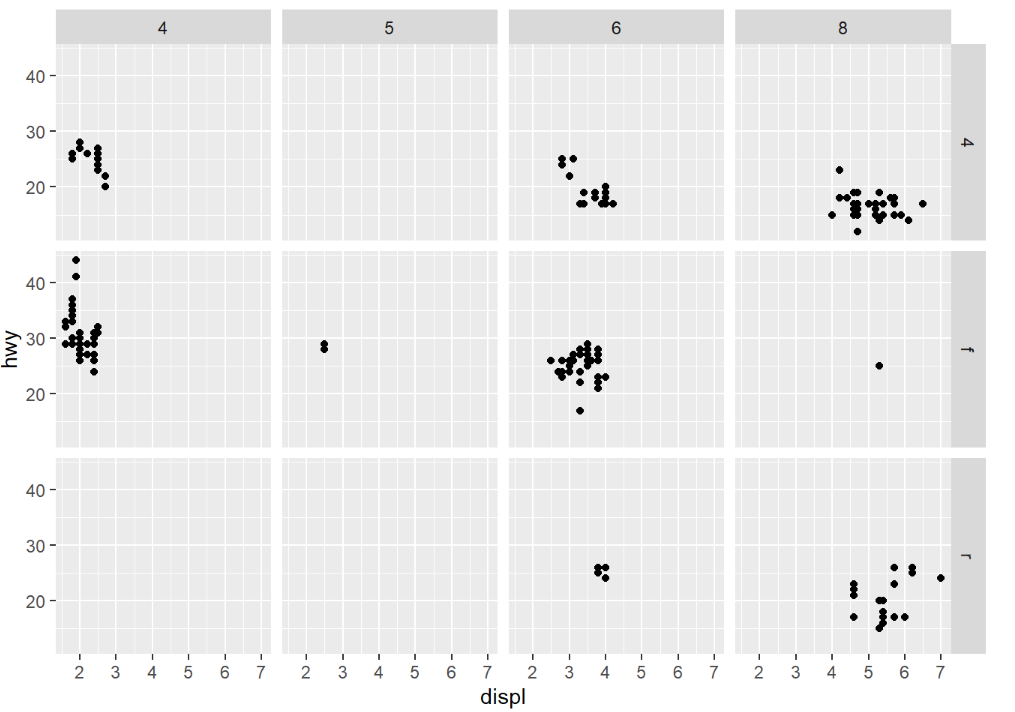#1

## [R語言]資料分析讀書心得02---Aesthetic Mappings

「圖表最重大的價值在於，它迫使我們注意到從沒預料會看到的東西。」---------John Tukey

``````library(tidyverse)
``````
``````ggplot(data=mpg)+
geom_point(mapping=aes(x=displ,y=hwy))
``````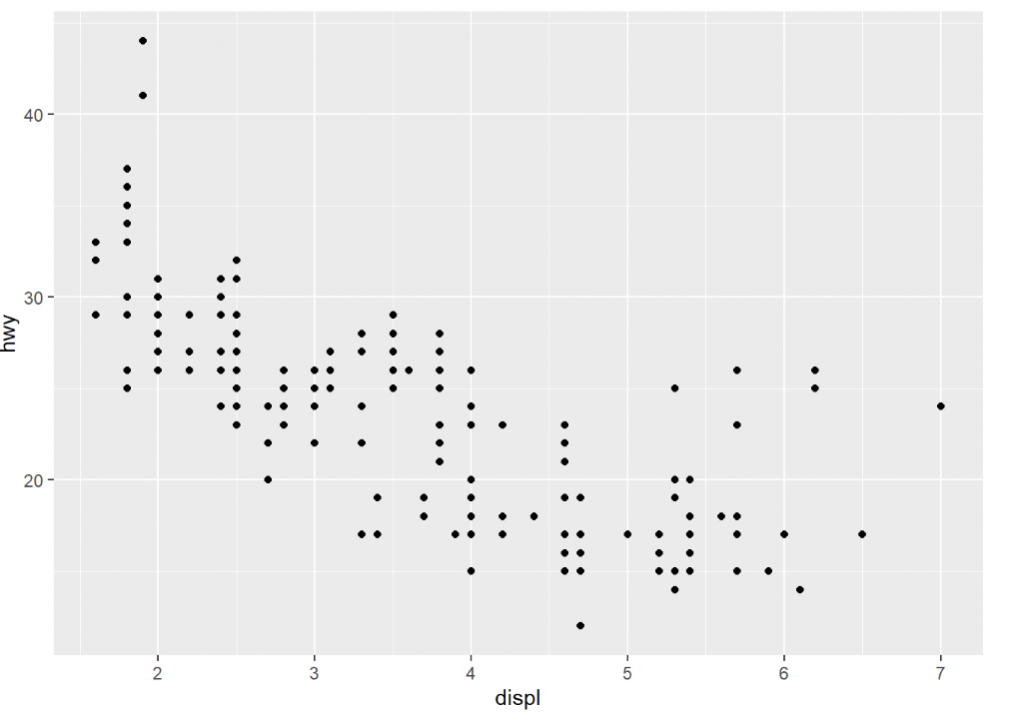``````glimpse(mpg)
``````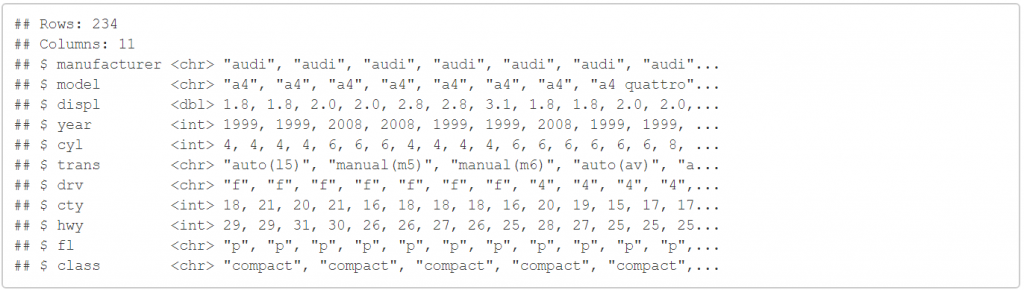``````ggplot(data=mpg)+
geom_point(mapping=aes(x=displ,y=hwy,color=class))
``````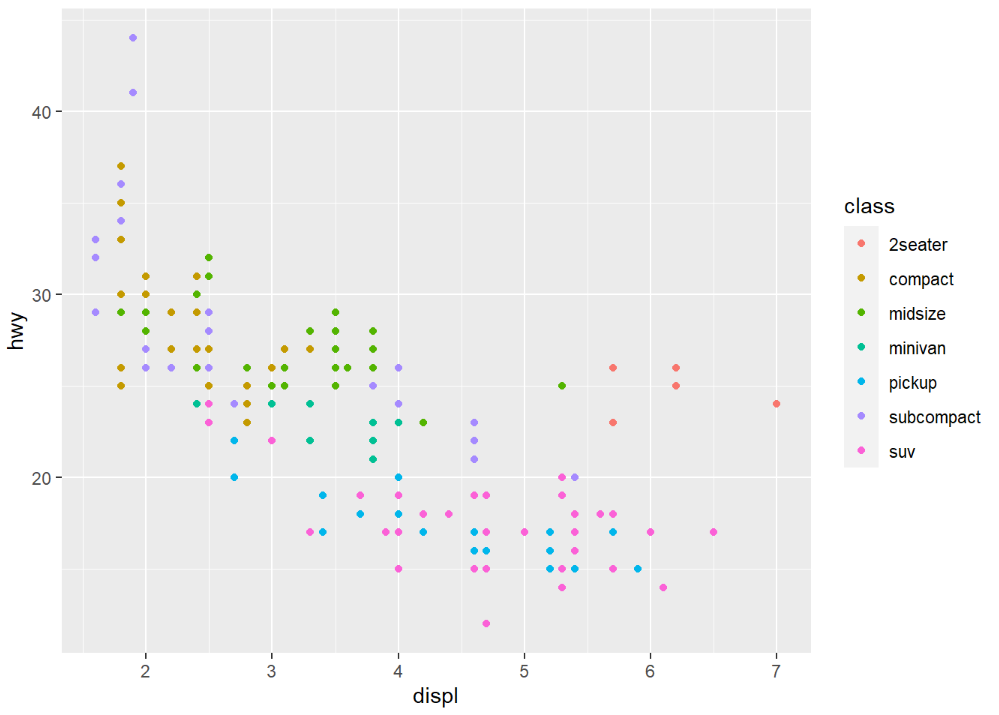``````ggplot(data=mpg)+
geom_point(mapping=aes(x=displ,y=hwy,size=class))
``````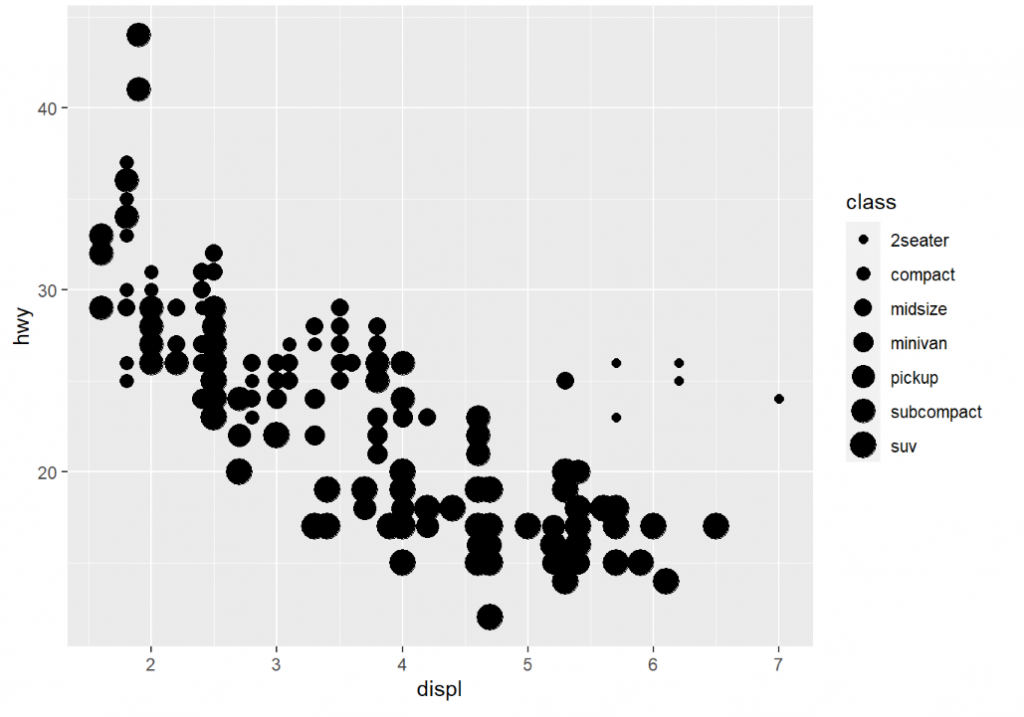``````ggplot(data=mpg)+
geom_point(mapping=aes(x=displ,y=hwy,alpha=class))
````````````ggplot(data=mpg)+
geom_point(mapping=aes(x=displ,y=hwy,shape=class))
``````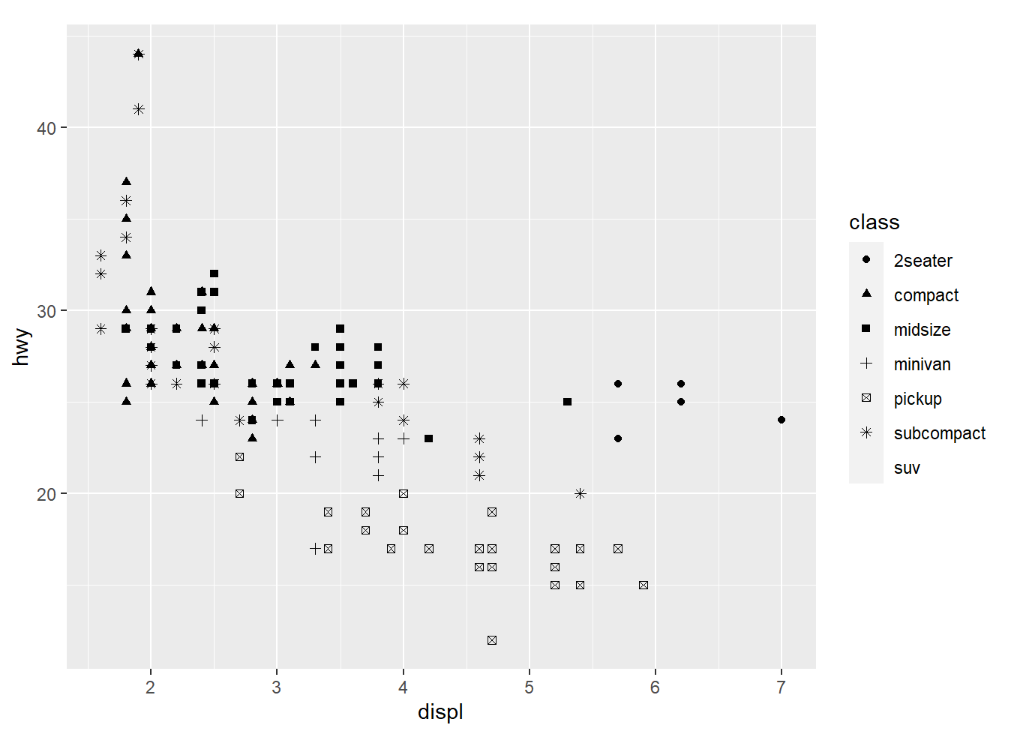``````ggplot(data=mpg)+
geom_point(mapping=aes(x=displ,y=hwy),color="blue")
``````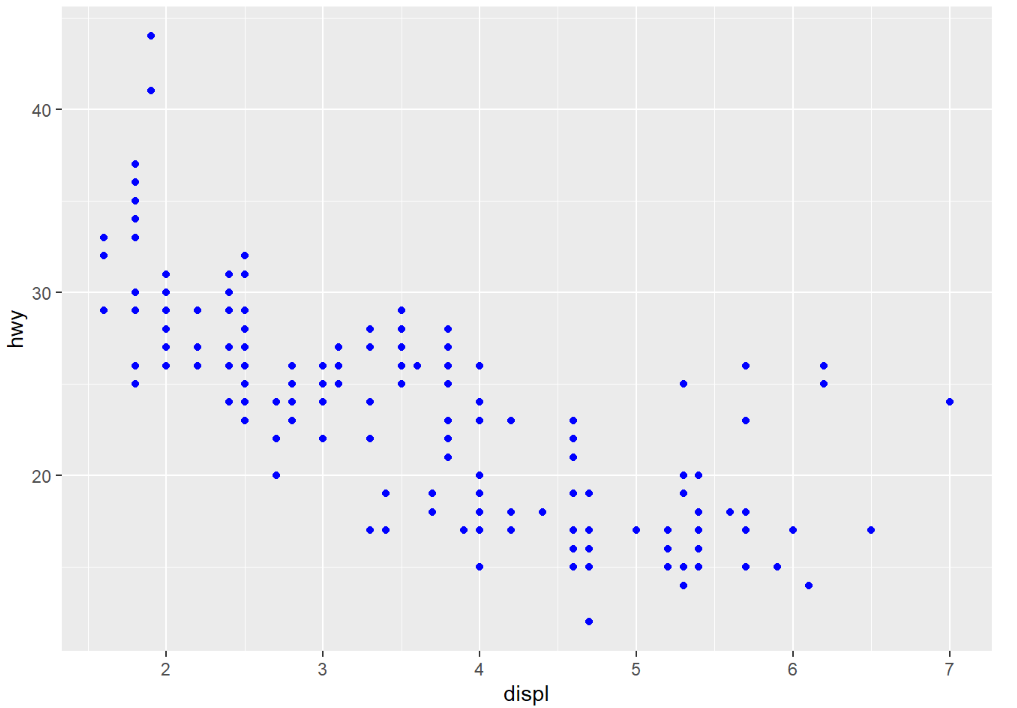``````ggplot(data=mpg)+
geom_point(mapping=aes(x=displ,y=hwy,color=displ<5))
``````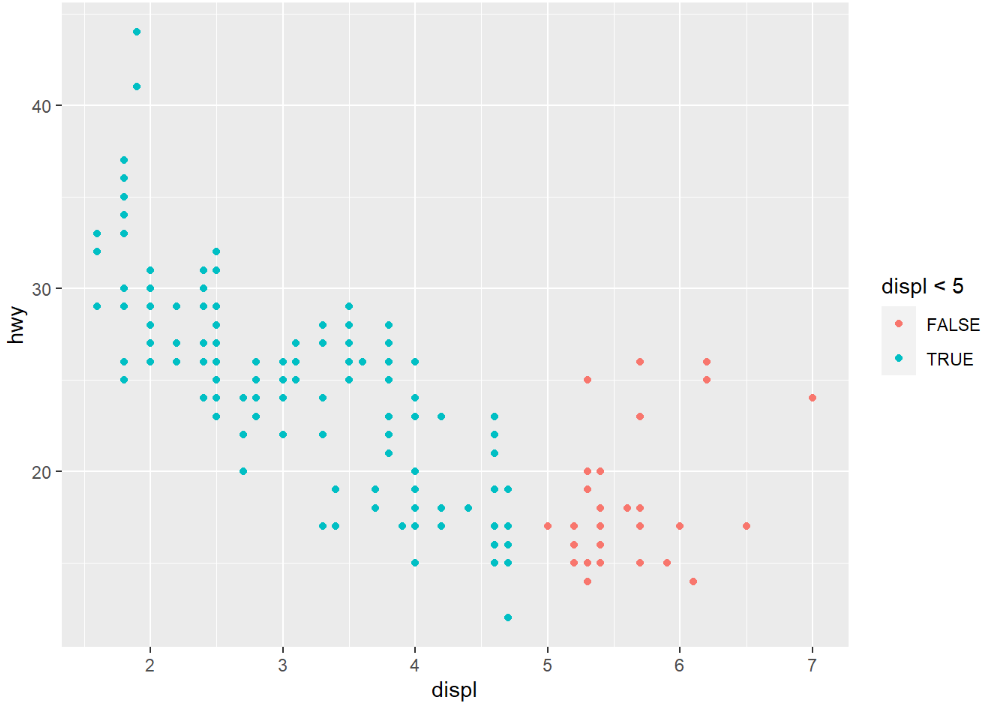``````ggplot(data=mpg)+
geom_point(mapping=aes(x=displ,y=hwy))+
facet_wrap(~class,nrow=2)
``````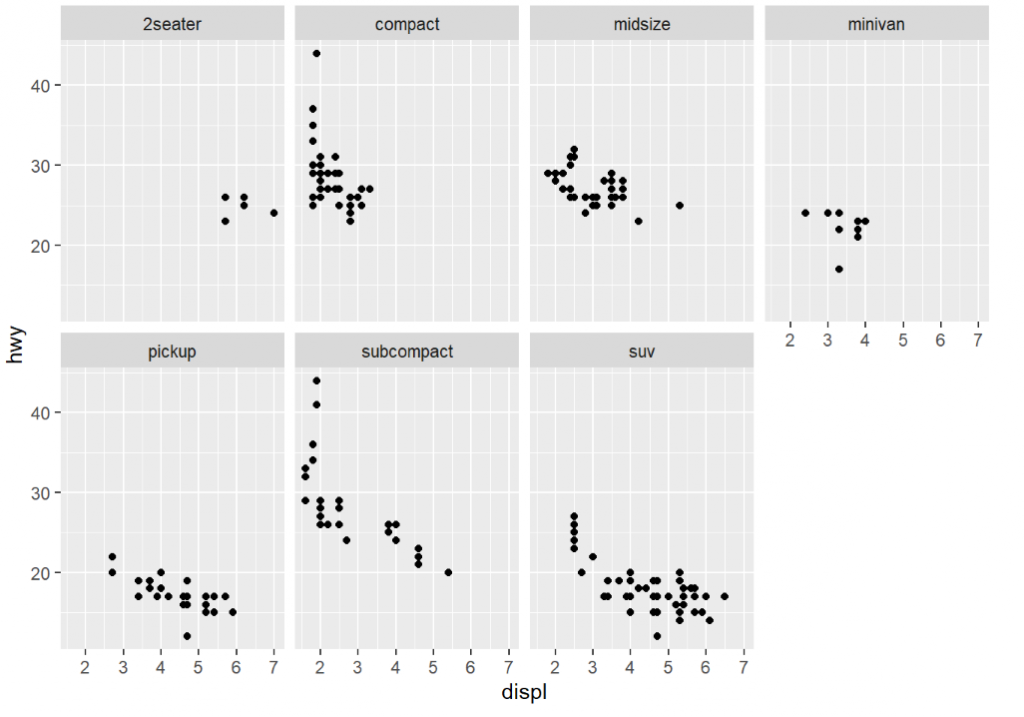``````ggplot(data=mpg)+
geom_point(mapping=aes(x=displ,y=hwy))+
facet_grid(drv~cyl)
``````